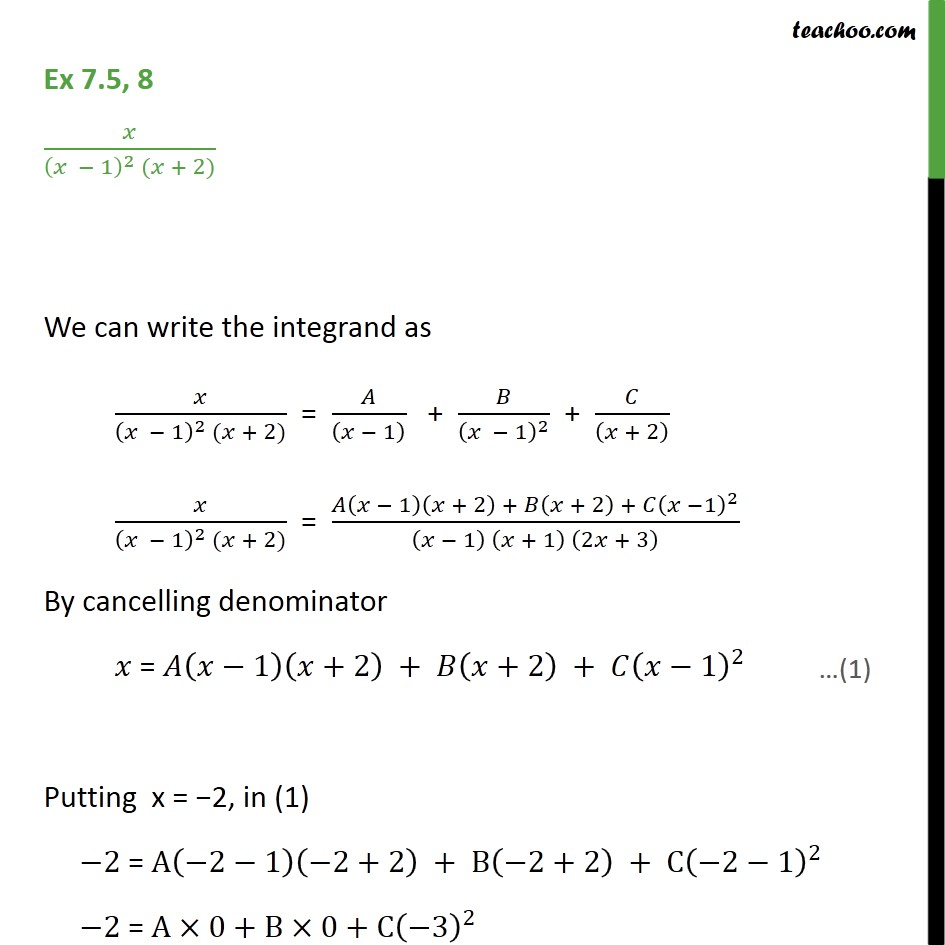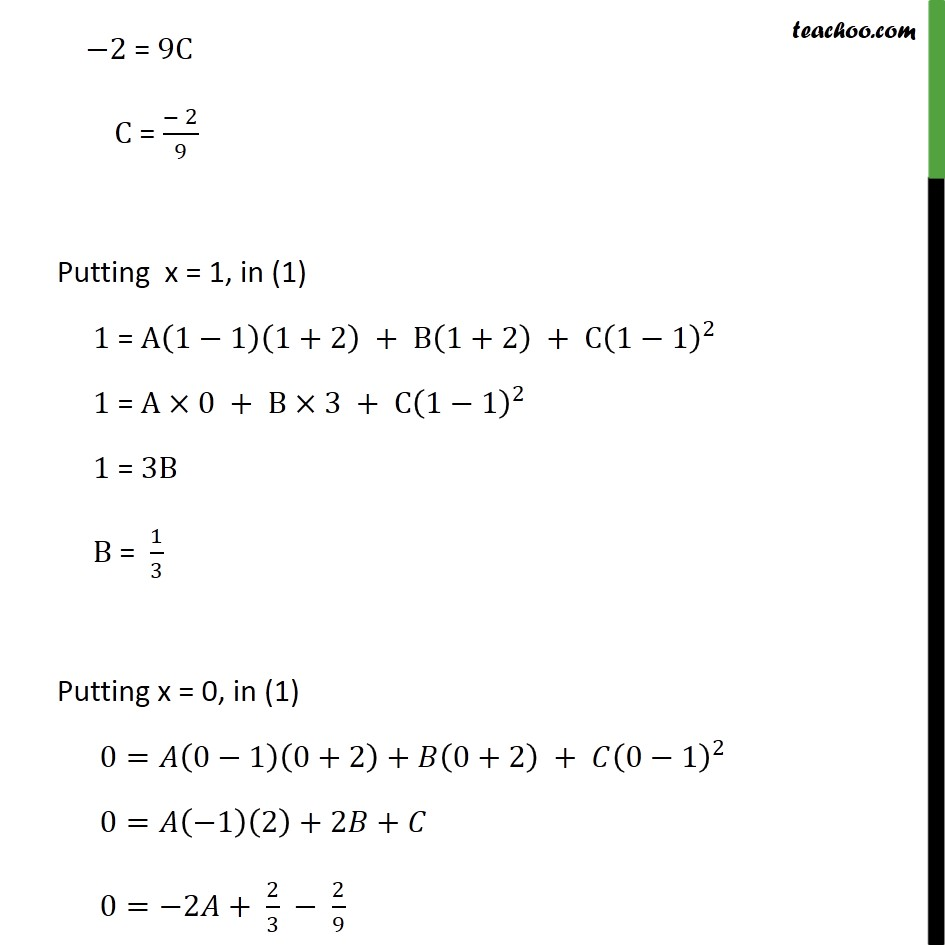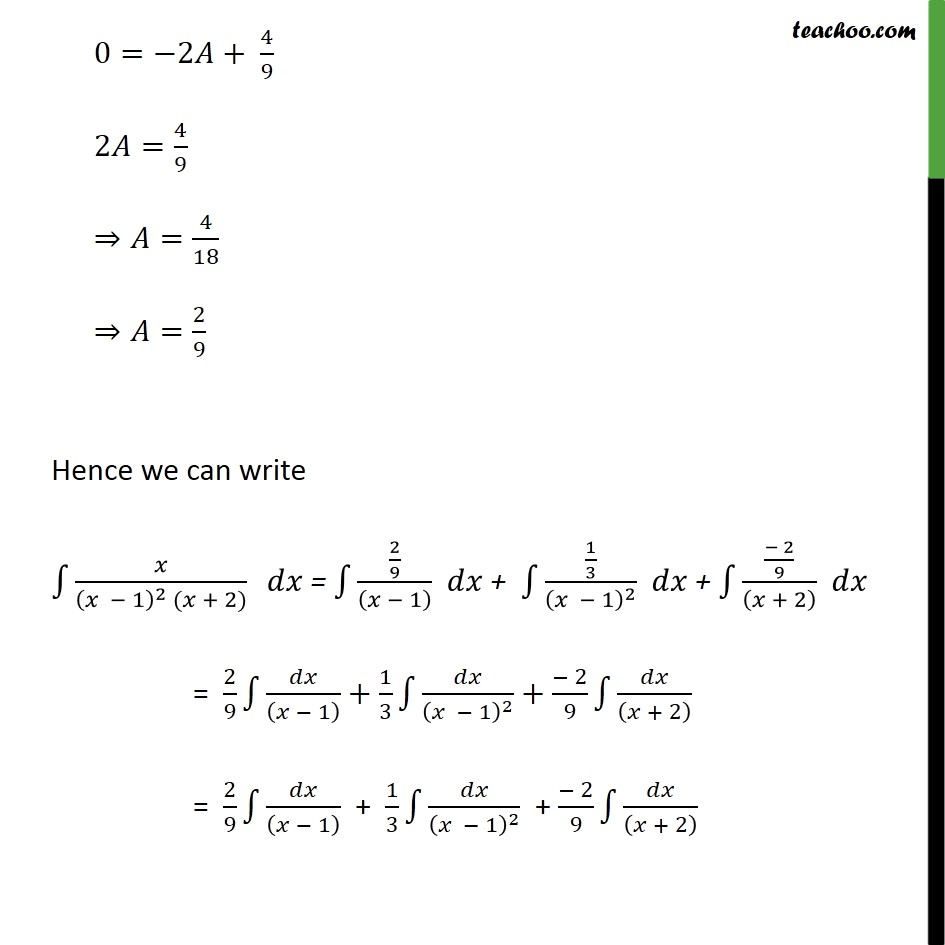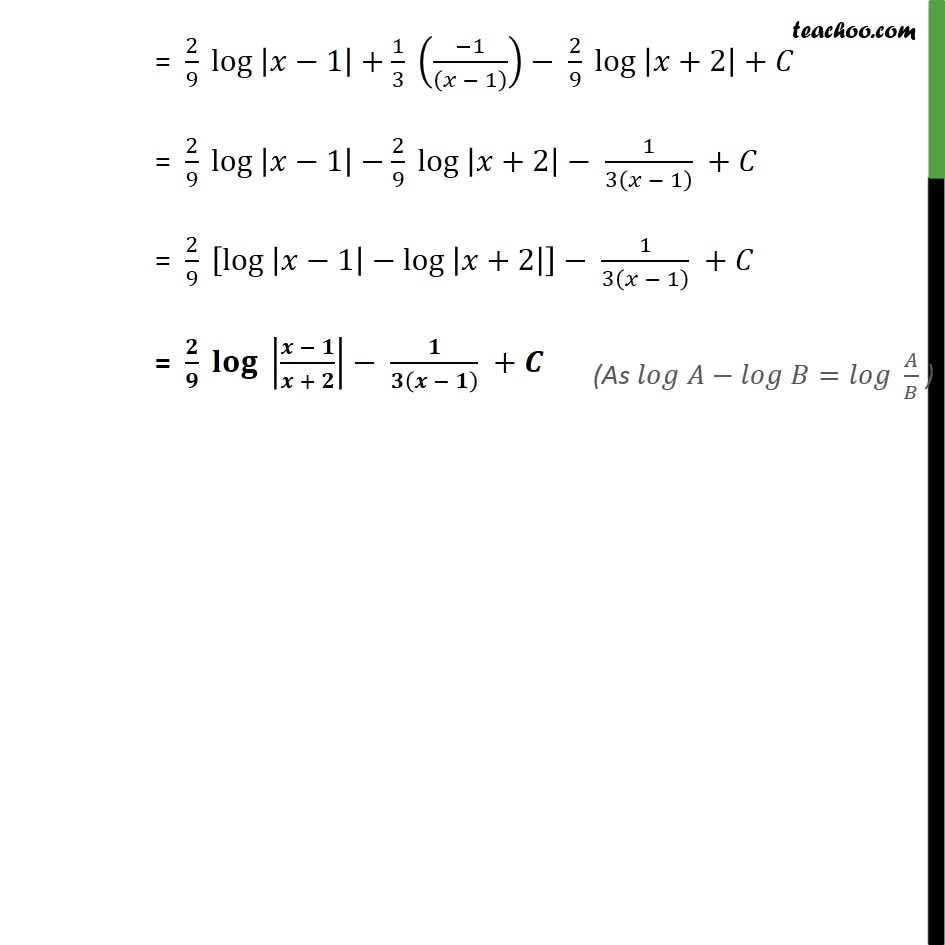Integration by partial fraction - Type 4

Chapter 7 Class 12 Integrals
Concept wiseLearn in your speed, with individual attention - Teachoo Maths 1-on-1 Class

### Transcript

Ex 7.5, 8 1 2 ( + 2) We can write the integrand as 1 2 ( + 2) = 1 + 1 2 + + 2 1 2 ( + 2) = 1 + 2 + + 2 + 1 2 1 + 1 2 + 3 By cancelling denominator = 1 +2 + +2 + 1 2 Putting x = 2, in (1) 2 = A 2 1 2+2 + B 2+2 + C 2 1 2 2 = A 0+B 0+C 3 2 2 = 9C C = 2 9 Putting x = 1, in (1) 1 = A 1 1 1+2 + B 1+2 + C 1 1 2 1 = A 0 + B 3 + C 1 1 2 1 = 3B B = 1 3 Putting x = 0, in (1) 0= 0 1 0+2 + 0+2 + 0 1 2 0= 1 2 +2 + 0= 2 + 2 3 2 9 0= 2 + 4 9 2 = 4 9 = 4 18 = 2 9 Hence we can write 1 2 ( + 2) = 2 9 1 + 1 3 1 2 + 2 9 + 2 = 2 9 1 + 1 3 1 2 + 2 9 + 2 = 2 9 1 + 1 3 1 2 + 2 9 + 2 = 2 9 log 1 + 1 3 1 1 2 9 log +2 + = 2 9 log 1 2 9 log +2 1 3 1 + = 2 9 log 1 log +2 1 3 1 + = + +